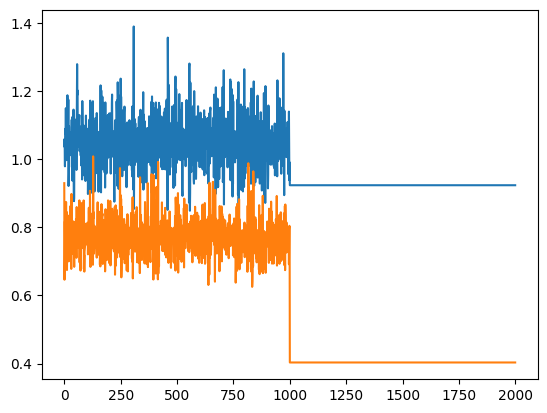# Example: Predator-Prey Model gives weird results when using num_chains > 2

Hello, I just tried the example, Example: Predator-Prey Model, with `adapt_step_size=False` and `num_chains=2` .

For `num_chains=1`, `adapt_step_size=True` works perfectly, but `num_chains > 2`, MCMC with `adapt_step_size=True` is peding forever, so I changed it to `False`. Also, I added the lines to enable multiple draws on the CPU.

``````numpyro.set_host_device_count(8)
print(jax.local_device_count())
``````

When I plot the trace of sigma, weird patterns are shown as follows

``````sigma = mcmc.get_samples()["sigma"]

# The number of samples = 1000.
plt.plot(sigma[:, 0])
plt.plot(sigma[:, 1])
plt.show()
``````Modification of ode options such as `rtol` , `atol` , `maxsteps` dosen’t solve the problem. As far as I know, `num_chains = 2` just performs sampling twice with difference random states (or starting potins for params maybe?). But It seems the second draw wasn’t performed. Any suggestions would be helpful.

Thank you.

I guess it’s pending because the ODE solver does not stop. I’m not sure why, given that we already specify maxsteps=1000. Could you let us know which command line you used to execute that example.